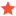BoxVisual3D AxisAngleRotation3D problemmpiccottiJunior MemberPosts: 2 Threads: 1 %%TYL_NUMTHANKEDLIKED%% Joined: Sep 2020 Reputation: 0 09-11-2020, 04:40 PM Good morning, using AxisAngleRotation3D I find problems in the inconsistency of rotations. The result of the rotations depends on the order in which I perform the rotations. To explain me better: To an object I first add a 90 degree Z rotation, then I add a 90 degree X rotation and the model performs the transformation I expect. By reversing the order of rotations, the model performs an unexpected transformation. I add the code to better understand the actions I perform. Code:```          AxisAngleRotation3D rot_X = new AxisAngleRotation3D(Ab3d.Common.Constants.XAxis., 90);          RotateTransform3D rotateTransform3D_X = new RotateTransform3D(rot_X, _selectedBoxModel.CenterPosition);          AddTransform(_selectedBoxModel, rotateTransform3D_X);          AxisAngleRotation3D rot_Y = new AxisAngleRotation3D(Ab3d.Common.Constants.YAxis., 90);          RotateTransform3D rotateTransform3D_Y = new RotateTransform3D(rot_Y , _selectedBoxModel.CenterPosition);          AddTransform(_selectedBoxModel, rotateTransform3D_Y);        private void AddTransform( Visual3D visual3D, Transform3D transform3D )        {            if ( visual3D.Transform == null )            {                visual3D.Transform = transform3D;                return;            }            var transform3DGroup = visual3D.Transform as Transform3DGroup;            if ( transform3DGroup == null )            {                // If there is already some other transformation, then create a new Transform3DGroup                transform3DGroup = new Transform3DGroup();                transform3DGroup.Children.Add(visual3D.Transform);                visual3D.Transform = transform3DGroup;            }            // Insert rotation as the first transformation - this way we are sure that it gets before any translations            if ( transform3DGroup.Children.Count > 0 )                transform3DGroup.Children.Insert(0, transform3D);            else                transform3DGroup.Children.Add(transform3D);        }``` Find ReplyjanovromJunior MemberPosts: 17 Threads: 8 %%TYL_NUMTHANKEDLIKED%% Joined: Aug 2019 Reputation: 0 09-11-2020, 06:10 PM Hi, It's actually correct behavior. You are combining rotations and that operation is not commutative (it's order dependent). Transform3D is also represented by a matrix and Transform3DGroup uses matrix multiplication, again that is not commutative operation. Find ReplyabenedikAdministratorPosts: 533 Threads: 8 %%TYL_NUMTHANKEDLIKED%% Joined: Sep 2009 Reputation: 4 09-14-2020, 09:54 PM As janovrom has explained, the order of rotations matters (expect if you rotate around the same axis then you can add or substitute the angles). Also the order of transformation matters: if you first do translation and then rotation, you will get different results as if you first rotate and then do a translation. Usually you first scale, then rotate and finally translate. But you can also simplify transformation with using StandardTransformaton3D. Note that because it is not possible to derive from Transform3D, you cannot simply assign StandardTransformaton3D to Transform property - instead you first create StandardTransformaton3D object and then assign its Transform property to Model3D.Transform or Visual3D.Transform - for example: Code:```var sphereVisual3D = new SphereVisual3D(); var standardTransform3D = new StandardTransform3D() {    RotateX    = 30,    RotateY    = 10,    TranslateX = 100 }; sphereVisual3D.Transform = standardTransform3D.Transform;``` With StandardTransformaton3D you can use TranslateX, TranslateY, TranslateY; ScaleX, ScaleY, ScaleZ; RotateX, RotateY, RotateZ properties. Andrej Benedik Find ReplympiccottiJunior MemberPosts: 2 Threads: 1 %%TYL_NUMTHANKEDLIKED%% Joined: Sep 2020 Reputation: 0 09-16-2020, 01:11 PM Thanks, it's a great solution for my application Michele Find Reply

Forum Jump:

 Users browsing this thread: 1 Guest(s)